# Excel COUNTBLANK and other functions to count empty cells

The tutorial discusses the syntax and basic uses of the COUNTBLANK function to count the number of blank cells in Excel.

In a couple of recent posts, we've discussed different ways to identify blank cells and highlight blanks in Excel. In some situations, however, you may want to know how many cells do not have anything in them. Microsoft Excel has a special function for this too. This tutorial will show you the fastest and most convenient methods to get the number of empty cells in a range as well as totally blank rows.

## Excel COUNTBLANK function

The COUNTBLANK function in Excel is designed to count empty cells in a specified range. It belongs to the category of Statistical functions and is available in all versions of Excel for Office 365, Excel 2019, Excel 2016, Excel 2013, Excel 2010, and Excel 2007.

The syntax of this function is very straightforward and requires just one argument:

COUNTBLANK(range)

Where range is the range of cells in which blanks are to be counted.

Here is an example of the COUNTBLANK formula in Excel in its simplest form:

`=COUNTBLANK(A2:D2)`

The formula, entered in E2 and copied down to E7, determines the number of empty cells in columns A through D in each row and returns these results: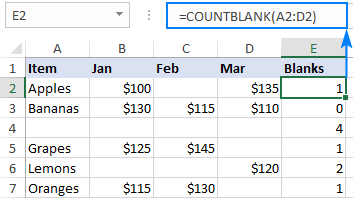Tip. To count non-blank cells in Excel, use the COUNTA function.

### COUNTBLANK function - 3 things to remember

To effectively use an Excel formula for counting blank cells, it is important to understand what cells the COUNTBLANK function considers as "blanks".

1. Cells that contain any text, numbers, dates, logical values, spaces or errors are not counted.
2. Cells containing zeros are considered non-blank and are not counted.
3. Cells containing formulas that return empty strings ("") are considered blank and are counted.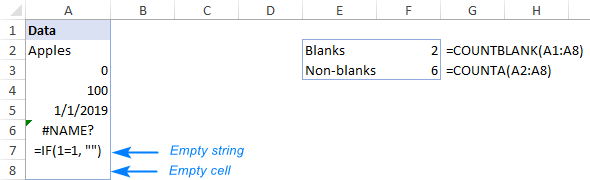Looking at the screenshot above, please notice that cell A7 containing a formula that returns an empty string is counted twice:

• COUNTBLANK considers a zero-length string as an empty cell because it appears blank.
• COUNTA treats a zero-length string as a non-empty cell because it actually contains a formula.

That may sound a bit illogical, but Excel does work this way :)

## How to count blank cells in Excel - formula examples

COUNTBLANK is the most convenient but not the only way to count empty cells in Excel. The following examples demonstrate a few other methods and explain which formula is best to be used in which scenario.

### Count blank cells in range with COUNTBLANK

Whenever you need to count blanks in Excel, COUNTBLANK is the first function to try.

For example, to get the number of empty cells in each row in the table below, we enter the following formula in F2:

`=COUNTBLANK(A2:E2)`

As we use relative references for the range, we can simply drag the formula down and the references will adjust automatically for each row, producing the following result: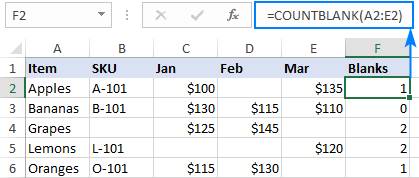### How to count blank cells in Excel using COUNTIFS or COUNTIF

Another way to count empty cells in Excel is to use the COUNTIF or COUNTIFS function or with an empty string ("") as the criteria.

In our case, the formulas would go as follows:

`=COUNTIF(B2:E2, "")`

Or

`=COUNTIFS(B2:E2, "")`

As you can see in the screenshot below, the results of COUNTIFS are exactly the same as those of COUNTBLANK, so which formula to use in this scenario is a matter of your personal preference.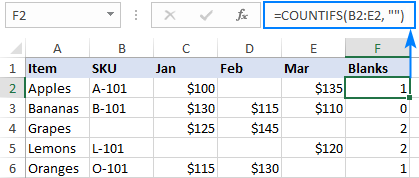### Count blank cells with condition

In a situation, when you want to count empty cells based on some condition, COUNTIFS is the right function to use as its syntax provides for multiple criteria.

For instance, to determine the number of cells that have "Apples" in column A and blanks in column C, use this formula:

`=COUNTIFS(A2:A9, "apples", C2:C9, "")`

Or input the condition in a predefined cell, say F1, and refer to that cell as the criteria:

`=COUNTIFS(A2:A9, F1, C2:C9, "")`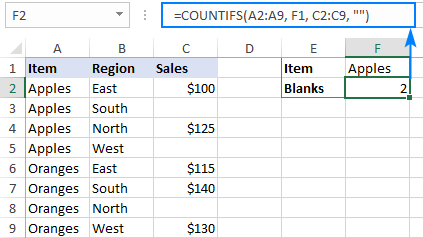### IF COUNTBLANK in Excel

In some cases, you may need not just count blank cells in a range, but take some action depending on whether there are any empty cells or not.

Although there is no built-in IF COUNTBLANK function in Excel, you can easily make your own formula by using the IF and COUNTBLANK functions together. Here's how:

• Check if the blanks count equals zero and put this expression in the logical test of IF:
`COUNTBLANK(B2:D2)=0`
• If the logical test evaluates to TRUE, output "No blanks".
• If the logical test evaluates to FALSE, output "Blanks".

The complete formula takes this shape:

`=IF(COUNTBLANK(B2:D2)=0, "No blanks", "Blanks")`

As the result, the formula identifies all the rows where one or more values are missing: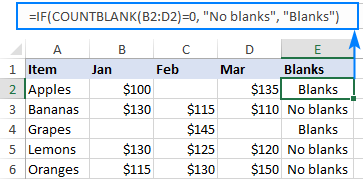Or you can run another function depending on the blanks count. For instance, if there are no empty cells in the range B2:D2 (i.e. if COUNTBLANK returns 0), then sum the values, otherwise return "Blanks":

`=IF(COUNTBLANK(B2:D2)=0, SUM(B2:D2), "Blanks")`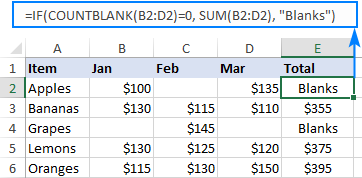### How to count blank rows in Excel

Supposing you have a table in which some rows contain information while other rows are totally blank. The question is - how do you get the number of rows that do not contain anything in them?

The easiest solution that comes to mind is to add a helper column and fill it with the Excel COUNTBLANK formula that finds the number of blank cells in each row:

`=COUNTBLANK(A2:E2)`

And then, use the COUNTIF function to find out in how many rows all the cells are blank. Since our source table contains 5 columns (A through E), we count the rows that have 5 empty cells:

`=COUNTIF(F2:F8, 5))`

Instead of "hardcoding" the number of columns, you can use the COLUMNS function to calculate it automatically:

`=COUNTIF(F2:F8, COLUMNS(A2:E2))`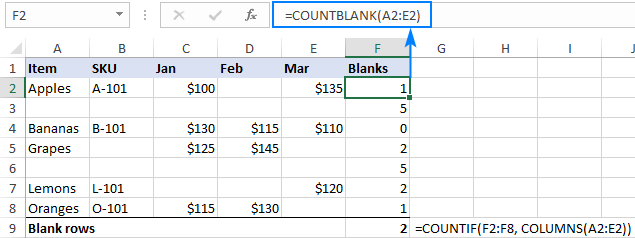If you do not want to mangle the structure of your beautifully designed worksheet, you can achieve the same result with a lot more complex formula that does not however require any helper columns nor even array entering:

`=SUM(--(MMULT(--(A2:E8<>""), ROW(INDIRECT("A1:A"&COLUMNS(A2:E8))))=0))`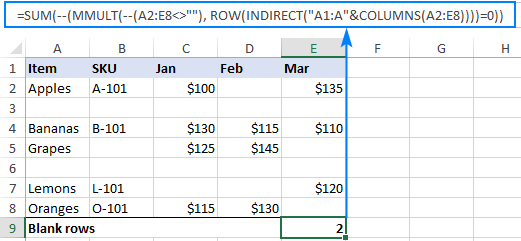Working from the inside out, here's what the formula does:

• First, you check the entire range for non-blank cells by using the expression like A2:E8<>"", and then coerce the returned logical values of TRUE and FALSE to 1's and 0's by using the double unary operator (--). The result of this operation is a two-dimensional array of ones (non-blanks) and zeros (blanks).
• The purpose of the ROW part is to generate a vertical array of numeric non-zero values, in which the number of elements equals to the number of columns of the range. In our case, the range consists of 5 columns (A2:E8), so we get this array: {1;2;3;4;5}
• The MMULT function calculates the matrix product of above arrays and produces a result like: {11;0;15;8;0;8;10}. In this array, the only thing that matters for us is 0 values that represent the rows where all cells are blank.
• Finally, you compare each element of the above array against zero, coerce TRUE and FALSE to 1 and 0, and then sum the elements of this final array: {0;1;0;0;1;0;0}. Keeping in mind that 1's correspond to blank rows, you get the desired result.

If the above formula seems too difficult for you to comprehend, you may like this one better:

`=SUM(--(COUNTIF(INDIRECT("A"&ROW(A2:A8) & ":E"&ROW(A2:A8)), "<>"&"")=0))`

Here, you use the COUNTIF function to find how many non-blank cells there are in each row, and INDIRECT "feeds" the rows to COUNTIF one by one. The result of this operation is an array like {4;0;5;3;0;3;4}. A check for 0, transforms the above array to {0;1;0;0;1;0;0} where 1's represent blank rows, so you just need to add them up.

### Count truly blank cells excluding empty strings

In all the previous examples, we were counting blank cells including those that only appear blank but, in reality, contain empty strings ("") returned by some formulas. In case you'd like to exclude zero-length strings from the result, you can use this generic formula:

ROWS(range) * COLUMNS(range) - COUNTA(range)

What the formula does is to multiply the number of rows by the number of columns to get the total of cells in the range, from which you subtract the number of non-blanks returned by COUNTA. As you may remember, the Excel COUNTA function considers empty strings as non-blank cells, so they won't be included in the final result.

For example, to determine how many absolutely empty cells there are in the range A2:A8, here's the formula to use:

`=ROWS(A2:A8) * COLUMNS(A2:A8) - COUNTA(A2:A8)`

The screenshot below shows the result: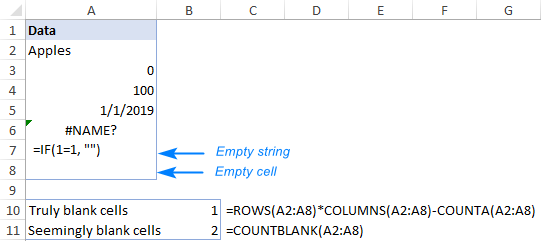That's how to count empty cells in Excel. I thank you for reading and hope to see you on our blog next week!

## You may also be interested in

1. there are multiple column ( 140 Column ) i am preparing data from 2008 to March 2023 . i am preparing sales data and want to now when was the first sales achieved and when did we raise last sales invoice

2. Alexander Trifuntov, I am really thankful for your guidance in the above! God bless you!

3. I want to count the blank cells in column P with respect to filled cells in Column F, I used countifs formula but the answer is not correct. Coul you please help me? Thanks

• Hi!
Have you tried the ways described in this blog post? If they don’t work for you, then please describe your task in detail, I’ll try to suggest a solution. To understand what you want to do, give an example of the source data and the desired result.

4. Hi, I am struggling to get the right formula; I am trying to count if one cell/column date is less than today which I think I am good with, & count the output based on another cell/column being false (the column currently contains tick boxes) - I am using =COUNTIFS(J4:J,">=B1",O4:O,"False") - however the answer I am getting isn't right, if anyone can advise that would be amazing.

• Sorry meant to say the date is greater than today's date (B1 contains today's date)

• Hello!
Pay attention to the syntax of the COUNTIFS function. Please try the following formula:

=COUNTIFS(J4:J1000,">="&B1,O4:O1000,"False")

• Thank you so much, yes it worked.. It was really starting to flummox me, it is nice to see id got my arguments right, just need to be more careful with my details. This site is awesome and especially your advice and support which you kindly provide. thank you

5. Hello, I'm trying to count the number of blanks in the column S, only if A, in the same row, has text entered. It's a continuing spreadsheet for inventory for my business, so the column S can possibly go on forever. I don't want to have to keep updating the formula every time I add a new item to our inventory. I'm so lost on this. :(

6. Hi, I'm trying to count the number of blank rows in a table on *Google Sheets* by modifying the provided (simpler) example formula:

=SUM(--(COUNTIF(INDIRECT("A"&ROW(A2:A8) & ":E"&ROW(A2:A8)), ""&"")=0)).

After much frustration why my modified formulas weren't working, I realized neither of the given examples work in Google Sheets at all...I recreated the exact example table in Google Sheets and in Excel and got 0 and 2 respectively.

It looks like the difference is how Excel and Google Sheets handle ROW() on ranges of cells (For example: =ROW(A1:A5)). Excel increments ROW() across the entire range. Google Sheets only returns ROW() on the first cell.

Any suggestions?
Thank you

• Hi Evan,

Since Google Sheets and Excel are different platforms, functions work differently. For example, to make ROW in Google Sheets process all rows in the range, you need to use ArrayFormula. But INDIRECT doesn't support ranges, only single cells.

So if you work in Google Sheets, use another formula – the one with the matrix:
=ARRAYFORMULA(SUM(--(MMULT(--(A2:E8<>""),ROW(INDIRECT("A1:A"&COLUMNS(A2:E8))))=0)))

Or, in case you have a small number of columns to process, you can try something like this:
=ROWS(A2:E8)-ROWS(FILTER(A2:E8,LEN(A2:A8)+LEN(B2:B8)+LEN(C2:C8)+LEN(D2:D8)+LEN(E2:E8)))

7. I had 30 cells in which I had a drop down "UNK, KNW" and depending on the data input drop down shall be selected. I need to provide max valu efor UNK and KNW list seperately. I had used formula =SUMPRODUCT(MAX((C24:C52="Knw")*(H24:H52))) and I am getting max valu ebut the moment I delete any of the cell values out of 30 "#Value" is played in out put cells. everytime all 30 cells will not be used for data entry but formaula needs to be freezed fro al 30 cells.

• Hi!
Unfortunately, I was unable to understand and reproduce your problem. Explain in more detail.

8. I am facing problem while using "counta'' function. It calculates total non-blank columns. But when I make a row blank intensionally, the "counta'' result remain the same. The scenario is "counta'' total is 6 and after making a column blank the total remain 6 (not 5).
Request for the solution.

• Hi!
I was unable to replicate your issue in my workbook. Read about the possible causes of your problem with the COUNTA function in this paragraph.

9. How can I count the number of blank cells above a cell?
e.g. If there is text in A1, 4 empty cells below it and I input the formula in cell A6, I would want the formula to return 4.

• Hello!
Perhaps your problem can be solved by the COUNTBLANK function, which can count empty cells. Please read the above article carefully.

10. To count a column with certain value else return a blank

• Hi!
I can't guess what you want to count - quantity or amount. Pay attention to the COUNTIFand SUMIF functions.

11. Hello,

how to count blank column from left to right,
if found non blank cell then stop there, ?????

• Hi!

=MATCH(FALSE,A1:AZ1="",0)-1

12. How to count blank cells in column B, but only for unique names in column A.

• Hello!
The formula below will do the trick for you:

=SUM(COUNTIFS(B2:B10,"",A2:A10,UNIQUE(A2:A10,FALSE,TRUE)))

To conditionally count blank cells, use the COUNTIFS function.

• That formula doesn't seem to be working for me. In column B I have 19 blanks and in column A I have 18 unique names, but the formula is generating 17. Could I be doing something wrong?
Thanks.

• Hello!
The formula I sent to you was created based on the description you provided in your first request. You have not specified which unique values you need. Formula extract values that occur only once. You may be looking for distinct values that occur more than once. Read carefully how the UNIQUE function works. Perhaps the function arguments need to be changed.

=SUM(COUNTIFS(B2:B8,"",A2:A8,UNIQUE(A2:A8,FALSE,FALSE)))

If this is not what you wanted, please describe the problem in more detail. Give an example of the source data and the expected result.

• Sorry, I thought I was making it easier the first time :) ...this is my problem.

In column A I have a list of names (that may be listed more than once), in column B I have volunteer hours associated with each name. I would like to generate a count of inactive volunteers by the hour cells that are blank (no hours=inactive) in column B but if it's a duplicate name in column A to only count the blank once.
I put the first formula in for this month (which all cells in column B are blank), which should return 18 but it returned 17.
Thank you!

• Hi!
I think the second formula will work for you. I already wrote it:

=SUM(COUNTIFS(B2:B8,””,A2:A8,UNIQUE(A2:A8,FALSE,FALSE)))

• Hi!
That one returns 19,so it counted the blank for the duplicate name both times.

13. How can I count blank cells in a specific column, with a row count that increases without having to change the Row range in the formula? I have 100 rows now and know how many blank cells are in the column. But now five more rows are added and the rows now go to 105. How to account for the increase without changing the formula?

I am using this formula now, but it counts ALL the rows and I need it to stop at 100, but account for rows inserted at the top. The COUNTIFS formula is suppose to be MTD check condition too, not sure if it is working either.

=COUNTBLANK(Cases!\$E:\$E) + COUNTIFS(Cases!C2, ">="&EOMONTH(TODAY(),-1)+1, Cases!C2, "<"&EOMONTH(TODAY(),0)+1)

Thanks,
Ron G

• Hello!
Your formula works with the entire column E. What does the “it counts ALL the rows and I need it to stop at 100” phrase mean? What do you want to calculate exactly? Your question is not entirely clear, please specify.

14. To count the number of blanks in each row for a particular column(E2) in all the sheets('17-Mar' till '12-Jun') in workbook in excel.

• Hello!
The COUNTBLANK function cannot count the number of empty cells using a 3D reference. Therefore, it must be used in the usual way, as described in this tutorial above.